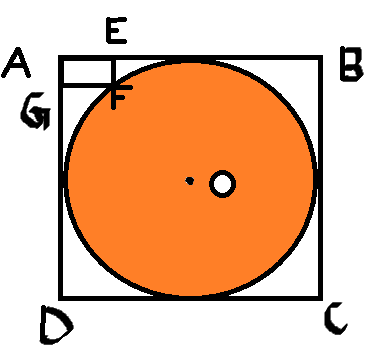# From rectangle to circle

Geometry Level 4In the above figure, $ABCD$ is a square and circle with centre $O$ is inscribed. In the corner, there is a rectangle $AEFG$ with $AG=10$ unit and $AE=20$ unit is inscribed. Find the area of circle.